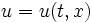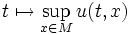# Max-decreasing trajectory

## Definition

Let$M$ be a manifold and$u = u(t,x)$ be a function$\R \times M \to \R$, where:

•$t$ denotes the time parameter, and varies in$\R$
•$x$ denotes the spatial parameter, and varies in$M$

In other words,$u$ is a trajectory (or path) in the space of all functions from$M$ to$\R$.

Then,$u$ is said to be max-reducing if the function:$t \mapsto \sup_{x \in M} u(t,x)$

is a monotone decreasing function.

The corresponding notion is of a min-increasing trajectory -- viz a trajectory where the minimum (or infimum) keeps increasing.